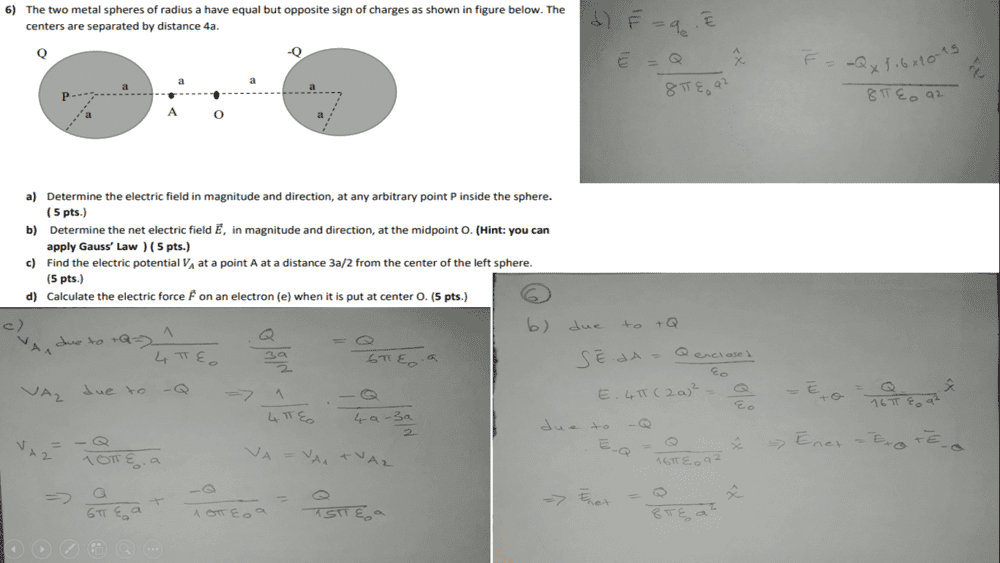# Could someone check the answers to these Gauss' Law questions?

wilywolie
Homework Statement:
physics 2
Relevant Equations:
Q=k.q1.q1/r2•denfaro

Cutter Ketch
b looks good although it’s bad form to not at least state what you are using for a surface in Gauss’ law

•denfaro and wilywolie
Cutter Ketch
I assume since you didn’t post anything you are happy with your answer for a

•wilywolie
wilywolie
I assume since you didn’t post anything you are happy with your answer for a
can you help me with case (a)? ı couldn't do that

•denfaro
Cutter Ketch
Actually, I’m starting to question whether b looks good. Gauss’ law is certainly true, but equating the integral to the field times the area assumes that the electric field is constant over the surface. In many problems you can make that assertion by symmetry, but is that true here?

•wilywolie and denfaro
wilywolie
Actually, I’m starting to question whether b looks good. Gauss’ law is certainly true, but equating the integral to the field times the area assumes that the electric field is constant over the surface. In many problems you can make that assertion by symmetry, but is that true here?
actually, ı am not sure for (b) that is why ı uploaded here, how can ı solve b or can you show me that. thank you.

Actually, I’m starting to question whether b looks good. Gauss’ law is certainly true, but equating the integral to the field times the area assumes that the electric field is constant over the surface. In many problems you can make that assertion by symmetry, but is that true here?

I am also unsure as to why an application of Gauss' law is necessary. It seems OP has used a spherical surface to determine the field strength at a point outside the sphere, which whilst being perfectly valid, reduces to a statement of Coulomb's law. Since any spherically symmetric distribution of charge is equivalent to a point charge at the centre (though granted, this can be shown with Gauss' law so might be why the problem-setter included it!).

•wilywolie
wilywolie
I am also unsure as to why an application of Gauss' law is necessary. It seems OP has used a spherical surface to determine the field strength at a point outside the sphere, which whilst being perfectly valid, reduces to a statement of Coulomb's law. Since any spherically symmetric distribution of charge is equivalent to a point charge at the centre (though granted, this can be shown with Gauss' law so might be why the problem-setter included it!).

What is the electric field inside a conducting material?

wilywolie
What is the electric field inside a conducting material?
integral E.ds / is it true ?

integral E.ds / is it true ?

That is the (negative of) the potential, when the limits are from infinity to your point.

If there were an electric field inside the conductor, charges would flow until the system reached equilibrium. So what must it be?

Cutter Ketch
I am also unsure as to why an application of Gauss' law is necessary. It seems OP has used a spherical surface to determine the field strength at a point outside the sphere, which whilst being perfectly valid, reduces to a statement of Coulomb's law. Since any spherically symmetric distribution of charge is equivalent to a point charge at the centre (though granted, this can be shown with Gauss' law so might be why the problem-setter included it!).

Maybe I jumped in too quickly here. I have no idea how to do this problem. One thing I am pretty sure of: it isn’t as simple as either his Gauss’ law solution or your equivalent Coulomb’s law assertion. The charges on the two spheres will attract. They will concentrate on the near faces. The electric field on the surfaces will not be uniform (limiting the utility of Gauss’ law) and they won’t act like a point source at the center (so no simple Coulomb’s law). In fact, you can say for absolute sure the field in the middle will be larger than these assumptions gives.

•etotheipi
Maybe I jumped in too quickly here. I have no idea how to do this problem. One thing I am pretty sure of: it isn’t as simple as either his Gauss’ law solution or your equivalent Coulomb’s law assertion. The charges on the two spheres will attract. They will concentrate on the near faces. The electric field on the surfaces will not be uniform (limiting the utility of Gauss’ law) and they won’t act like a point source at the center (so no simple Coulomb’s law). In fact, you can say for absolute sure the field in the middle will be larger than these assumptions gives.

I think I jumped in too quickly too. You're absolutely right, there's no spherical symmetry of charge for either of the spheres in this system.

Cutter Ketch
You know, given the hint about using Gauss’ law, it seems like the creator of the question might have made the same mistake?

•etotheipi